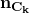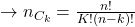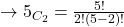## Problem 2.4 You would like to evaluate the probability of success for testing a batch of n processors. To start out, let’s assume that if th

Question

Problem 2.4 You would like to evaluate the probability of success for testing a batch of n processors. To start out, let’s assume that if there is a problem with the batch, exactly 1 out of the n processors are defective. You are willing to test only k of the processors (due to budget or times constraints). (a) How many ways are there of testing k out of n processors

in progress 0
3 weeks 2021-09-05T08:33:36+00:00 1 Answers 0 views 0

The answer is “Step-by-step explanation:For Example:

if n=5 and k=2